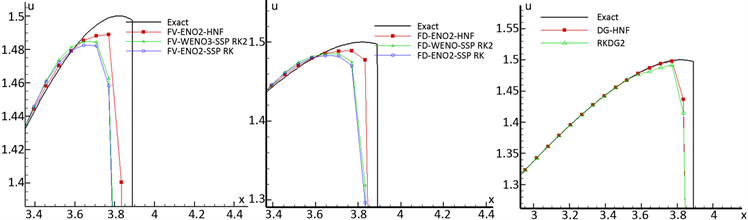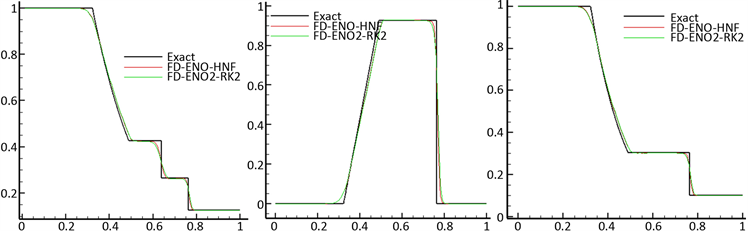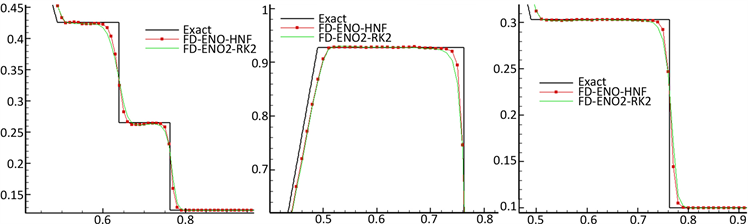﻿ 一种高阶数值通量的探讨

# 一种高阶数值通量的探讨An Approach of High Order Numerical Flux

Abstract: Referring to the construction idea of the MUSCL scheme, we present a construction method for high-order numerical fluxes. The higher order numerical flux is applied to the finite volume (dif-ference) ENO, WENO and DG schemes to obtain single step higher order Semi-Lagrangian scheme. For the one-dimensional Euler equations, this paper presents a new feature line processing scheme in the feature space, which solves the difficulty that the Semi-Lagrangian method is difficult to gen-eralize to multidimensional. Numerical experiments show that the new format is smaller than the original format, the efficiency is higher, and the simulation effect on the shock wave is also greatly improved.

1. 引言

1959年，Godunov首次提出以Riemann问题解为基础来构造网格物理量均值的Godunov格式，它有效地解决了所有内部状态变量的局部黎曼问题  。随后，Van Leer首次讨论了二阶Godunov格式的构造得到了MUSCL (Monotone Upwind Scheme for Conservation Laws)格式，这个格式包括了保单调性的插值和利用Riemann解得到的迎风通量，提高了数值精度和分辨率 。MUSCL格式已经被许多人研究   ，并被广泛用于科学和工程问题的模拟 。

1959年，Wiin-Nielsen提出了一种修正轨迹方法 ，随后Sawyer将其发展为Semi-Lagrangian方法 。该方法具有固定的(欧拉)数值网格，根据Riemann不变量沿特征线不变的性质，从而推进每个时间步，使得时间步长不受CFL条件的限制，减少计算工作量和数值扩散 。Semi-Lagrangian方法成功地推广到了有限差分，有限元和谱方法，并广泛应用于天气预报，海洋学模型的数值模拟和线性对流的建模  。1982年，Robert将半隐式(SI)和半拉格朗日(SL)这两种方法相结合，在NWP模型中实现了显著的效率增益，为许多环境应用产生了许多有效的SISL模型 。1999年，Strain将水平集方法和半拉格朗日方法相结合，能有效、自适应和模块化求解几何运动界面问题  。2006年，Bonaventura和Restelli等人将DG方法与半拉格朗日方法相结合为SLDG，能有效地融合了两种方法优点，又避免了缺点 ，对于shallow water方程模拟，SLDG具有较高的精度和有效性，并可自动选择局部逼近程度 。2010年，Qiu等人将Strang时间分裂与空间高阶WENO重构相结合，应用于Semi-Lagrangian方法来求解Vlasov方程，比使用显式时间步长的Vlasov-Poisson系统的欧拉公式计算更高效，更灵活 。

2. 二阶数值通量的推导

${u}_{t}+f{\left(u\right)}_{x}=0,\text{\hspace{0.17em}}\text{\hspace{0.17em}}\left(x,t\right)\in \left[a,b\right]×\left[0,T\right],$ (1)

$u\left(x,0\right)={u}_{0}\left(x\right).$ (2)

${p}_{j}\left(x\right)=u\left(x,{t}_{n}\right)={u}_{j+1/2}^{-}\left({t}_{n}\right)+\frac{{u}_{j+1/2}^{-}\left({t}_{n}\right)-{u}_{j-1/2}^{+}\left({t}_{n}\right)}{{h}_{j}}\left(x-{x}_{j+1/2}\right),$ (3)

$\frac{\text{d}}{\text{d}s}X\left(x,t;t+s\right)=\frac{\partial }{\partial u}f\left(u\left(X\left(x,t;t+s\right),t+s\right)\right)=h\left(X\left(x,t;t+s\right),t+s\right),$ (4)

$X={x}_{j+1/2}-\Delta sh\left(X\left({x}_{j+1/2},{t}_{n};{t}_{n}+s\right),{t}_{n}+s\right),$ (5)

$u\left(X,{t}_{n}\right)={u}_{j+1/2}^{-}\left({t}_{n}\right)+\frac{{u}_{j+1/2}^{-}\left({t}_{n}\right)-{u}_{j-1/2}^{+}\left({t}_{n}\right)}{{h}_{j}}\Delta sh\left(X\left({x}_{j+1/2},{t}_{n};{t}_{n}+s\right),{t}_{n}+s\right).$ (6)

${\stackrel{^}{f}}_{j+1/2}=\frac{f\left(u\left(X,{t}_{n}\right)\right)+f\left(u\left({x}_{j+1/2},{t}_{n}\right)\right)}{2}.$ (7)

3. 二阶数值通量在DG格式的应用

${\iint }_{{\Omega }_{j}}\left({\partial }_{t}{u}_{h}+{\partial }_{x}f\left({u}_{h}\right)\right)\phi \left(x\right)\text{d}x\text{d}t=0,$ (8)

$\begin{array}{l}{\int }_{{x}_{j-1/2}}^{{x}_{j+1/2}}\left({u}_{h}\left(x,{t}_{n+1}\right)-{u}_{h}\left(x,{t}_{n}\right)\right)\phi \left(x\right)\text{d}x+{\int }_{{t}_{n}}^{{t}_{n+1}}f\left({u}_{h}\left({x}_{j+1/2},t\right)\right)\phi \left({x}_{j+1/2}\right)\text{d}t\\ \text{ }-{\int }_{{t}_{n}}^{{t}_{n+1}}f\left({u}_{h}\left({x}_{j-1/2},t\right)\right)\phi \left({x}_{j-1/2}\right)\text{d}t-{\iint }_{{\Omega }_{j}}f\left({u}_{h}\right){\partial }_{x}\phi \left(x\right)\text{d}x\text{d}t=0,\end{array}$ (9)

${\int }_{{I}_{j}}{u}_{h}\left(x,0\right)\phi \left(x\right)\text{d}x={\int }_{{I}_{j}}{u}_{0}\left(x\right)\phi \left(x\right)\text{d}x.$ (10)

${u}_{j}^{0}\left({t}_{n+1}\right)={u}_{j}^{0}\left({t}_{n}\right)-\frac{\Delta t}{\Delta x}\left({\stackrel{^}{f}}_{j+1/2}-{\stackrel{^}{f}}_{j-1/2}\right),$ (11)

${u}_{j}^{1}\left({t}_{n+1}\right)={u}_{j}^{1}\left({t}_{n}\right)-\frac{3\Delta t}{\Delta x}\left({\stackrel{^}{f}}_{j+1/2}+{\stackrel{^}{f}}_{j-1/2}\right)+\frac{3}{\Delta x}{\iint }_{{\Omega }_{j}}f\left({u}_{h}\right){\partial }_{x}{\phi }_{1}\left(x\right)\text{d}x\text{d}t.$ (12)

$K=\frac{\Delta t}{\Delta x}\left({\int }_{{I}_{j}}f\left({u}_{h}\left(x,{t}_{n+1}\right)\right)\text{d}x+{\int }_{{I}_{j}}f\left({u}_{h}\left(x,{t}_{n}\right)\right)\text{d}x\right),$ (13)

$K=\Delta t\left[f\left({u}_{j}^{0}\left({t}_{n+1}\right)\right)+\frac{1}{2}\left(f\left({u}_{h}\left({x}_{j+1/2},{t}_{n}\right)\right)+f\left({u}_{h}\left({x}_{j-1/2},{t}_{n}\right)\right)\right)\right].$ (14)

4. 一维Euler方程组上的二阶数值通量的推导

${u}_{t}+{\left[F\left(u\right)\right]}_{x}=0,$ (15)

$u={\left(\rho ,\rho u,\rho E\right)}^{\text{T}},\text{\hspace{0.17em}}\text{\hspace{0.17em}}F\left(u\right)={\left(\rho u,\rho {u}^{2}+p,\left(\rho E+p\right)u\right)}^{\text{T}}$

${\lambda }_{1}=u-c,{\lambda }_{2}=u,{\lambda }_{3}=u+c,$

${u}_{t}+{\left(R\Lambda {R}^{-1}u\right)}_{x}=0,$

${p}_{j}\left(x,{t}_{n}\right)=u\left(x,{t}_{n}\right)={u}_{j+1/2}^{-}\left({t}_{n}\right)+\frac{{u}_{j+1/2}^{-}\left({t}_{n}\right)-{u}_{j-1/2}^{+}\left({t}_{n}\right)}{{h}_{j}}\left(x-{x}_{j+1/2}\right),$

$\frac{\text{d}}{\text{d}s}{X}_{k}\left(x,t;t+s\right)=-{\lambda }_{k}\left({X}_{k}\left(x,t;t+s\right),t+s\right),k=1,2,3$ (16)

${W}_{k}\left({X}_{k},{t}_{n}\right)={R}^{-1}{u}_{k}\left({X}_{k},{t}_{n}\right)={\left({W}_{k1},{W}_{k2},{W}_{k3}\right)}^{\text{T}}$ ，则 $W\left({x}_{j+1/2},{t}_{n+1}\right)={\left({W}_{11},{W}_{22},{W}_{33}\right)}^{\text{T}}$

${\stackrel{^}{F}}_{j+1/2}=\frac{F\left(u\left({x}_{j+1/2},{t}_{n}\right)\right)+F\left(u\left({x}_{j+1/2},{t}_{n+1}\right)\right)}{2}=\frac{R\Lambda \left(W\left({x}_{j+1/2},{t}_{n}\right)+W\left({x}_{j+1/2},{t}_{n+1}\right)\right)}{2}.$ (17)

5. 数值算例

${u}_{j}^{n+1}={u}_{j}^{n}-\frac{\Delta t}{\Delta x}\left({\stackrel{^}{f}}_{j+1/2}-{\stackrel{^}{f}}_{j-1/2}\right),$ (18)Table 1. Numerical error table for single-step second-order ENO, DG format and original format at t = 0.5Table 2. Numerical error meter of third order WENO scheme in = 0.5 FVWENO3-HNFFigure 1. The local comparison of real and numerical solutions in t = 1.5

$\left(\rho ,u,p\right)=\left\{\begin{array}{l}\left(1,0,1\right),\text{\hspace{0.17em}}\text{\hspace{0.17em}}\text{\hspace{0.17em}}\text{\hspace{0.17em}}\text{\hspace{0.17em}}\text{\hspace{0.17em}}\text{\hspace{0.17em}}\text{\hspace{0.17em}}\text{\hspace{0.17em}}\text{\hspace{0.17em}}\text{\hspace{0.17em}}\text{\hspace{0.17em}}x\le 0.5\\ \left(0.125,0,0.1\right),\text{\hspace{0.17em}}\text{\hspace{0.17em}}\text{\hspace{0.17em}}x>0.5\end{array}$Figure 2. The overall comparison between the true solution and the numerical solution in t = 0.15(a) 密度 (b) 速度 (c) 压力

Figure 3. The local comparison between the true solution and the numerical solution in t = 0.15

FD-ENO2-HNF格式所消耗的CPU时间为282毫秒(ms)，标准FD-ENO2格式所消耗的CPU时间为400毫秒(ms)，FD-ENO-HNF格式比标准FD-ENO2格式节约了29.5%左右。

6. 总结

NOTES

*通讯作者

 Bidadi, S. and Rani, S.L. (2014) Quantification of Numerical Diffusivity due to TVD Schemes in the Advection Equation. Journal of Computational Physics, 261, 65-82.
https://doi.org/10.1016/j.jcp.2013.12.011

 水鸿寿. 一维流体力学差分方法[M]. 北京: 国防大学出版社, 1998: 15-26.

 Hou, J., Liang, Q., Zhang, H. and Hinkelmann, R. (2015) An Efficient Unstructured MUSCL Scheme for Solving the 2D Shallow Water Equations. Environmental Modelling & Software, 66, 131-152.
https://doi.org/10.1016/j.envsoft.2014.12.007

 Sun, D. (2018) Performance Study of MUSCL Schemes Based on Different Numerical Fluxes. Wireless Personal Communications, 102, 1763-1772.
https://doi.org/10.1007/s11277-017-5234-8

 Sohn, S.I. (2005) A New TVD-MUSCL Scheme for Hyperbolic Conservation Laws. Computers & Mathematics with Applications, 50, 231-248.
https://doi.org/10.1016/j.camwa.2004.10.047

 Wiin-Nielsen, A. (1959) On the Application of Trajectory Methods in Numerical Forecasting. Tellus, 11, 180-196.
https://doi.org/10.3402/tellusa.v11i2.9300

 Sawyer, J.S. (1963) A Semi-Lagrangian Method of Solving the Vorticity Advection Equation. Tellus, 15, 336-342.
https://doi.org/10.3402/tellusa.v15i4.8862

 Huang, C.-S., Arbogast, T. and Hung, C.-H. (2016) A Semi-Lagrangian Finite Difference WENO Scheme for Scalar Nonlinear Conservation Laws. Journal of Computational Physics, 322, 559-585.
https://doi.org/10.1016/j.jcp.2016.06.027

 Piao, X., Kim, P. and Kim, D. (2018) One-Step, L(α)-Stable Temporal Integration for the Backward Semi-Lagrangian Scheme and Its Application in Guiding Center Problems. Journal of Computational Physics, 366, 327-340.
https://doi.org/10.1016/j.jcp.2018.04.019

 Strain, J. (1999) Semi-Lagrangian Methods for Level Set Equations. Journal of Computational Physics, 151, 498-533.
https://doi.org/10.1006/jcph.1999.6194

 Tumolo, G., Bonaventura, L. and Restelli, M. (2013) A Semi-Implicit, Semi-Lagrangian, p-Adaptive Discontinuous Galerkin Method for the Shallow Water Equations. Journal of Computational Physics, 232, 46-67.
https://doi.org/10.1016/j.jcp.2012.06.006

 Robert, A. (1982) A Semi-Lagrangian and Semi-Implicit Numerical Integration Scheme for the Primitive Meteorological Equations. Journal of the Meteorological Society of Japan, 60, 319-325.
https://doi.org/10.2151/jmsj1965.60.1_319

 Restelli, M., Bonaventura, L. and Sacco, R. (2006) A Semi-Lagrangian Discontinuous Galerkin Method for Scalar Advection by Incompressible Flows. Journal of Compu-tational Physics, 216, 195-215.
https://doi.org/10.1016/j.jcp.2005.11.030

 Qiu, J.-M. and Christlieb, A. (2010) A Conservative High Order Semi-Lagrangian WENO Method for the Vlasov Equation. Journal of Computational Physics, 229, 1130-1149.
https://doi.org/10.1016/j.jcp.2009.10.016

 Kwatra, N., Su, J., Grétarsson, J.T. and Fedkiw, R. (2009) A Method for Avoiding the Acoustic Time Step Restriction in Compressible Flow. Journal of Computational Physics, 228, 4146-4161.
https://doi.org/10.1016/j.jcp.2009.02.027

 Lentine, M., Grétarsson, J.T. and Fedkiw, R. (2011) An Uncondi-tionally Stable Fully Conservative Semi-Lagrangian Method. Journal of Computational Physics, 230, 2857-2879.
https://doi.org/10.1016/j.jcp.2010.12.036

 Li, S. and Xiao, F. (2007) CIP/Multi-Moment Finite Volume Method for Euler Equations: A Semi-Lagrangian Characteristic Formulation. Journal of Computational Physics, 222, 849-871.
https://doi.org/10.1016/j.jcp.2006.08.015

 吴浪. 双曲守恒律方程的高阶半拉格朗日方法[D]: [博士学位论文]. 哈尔滨: 哈尔滨工业大学, 2015.

Top